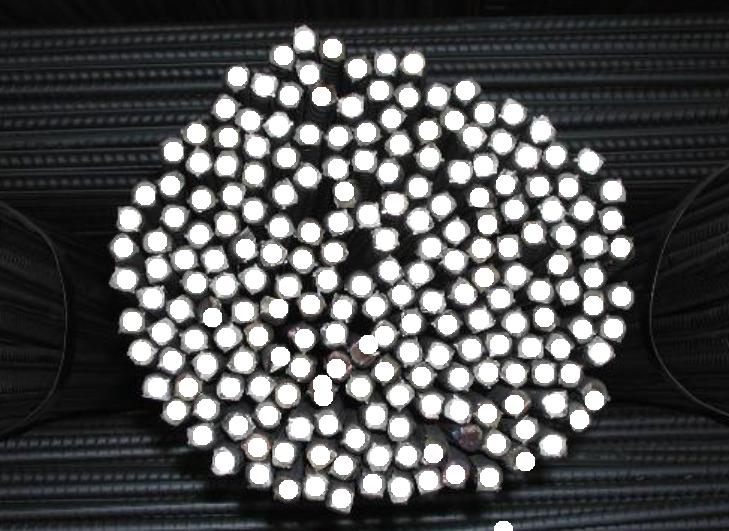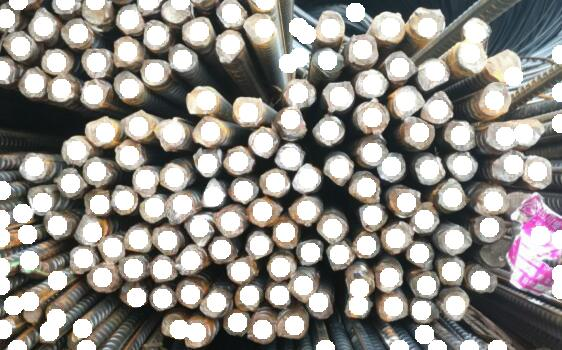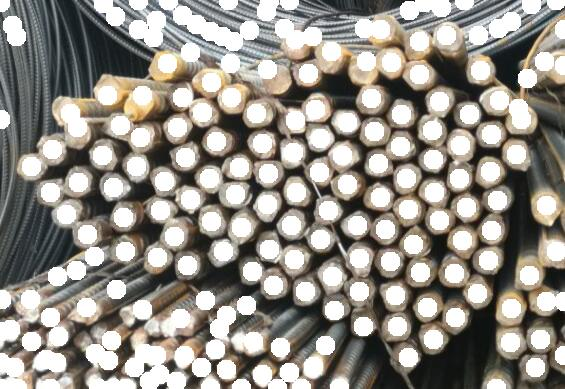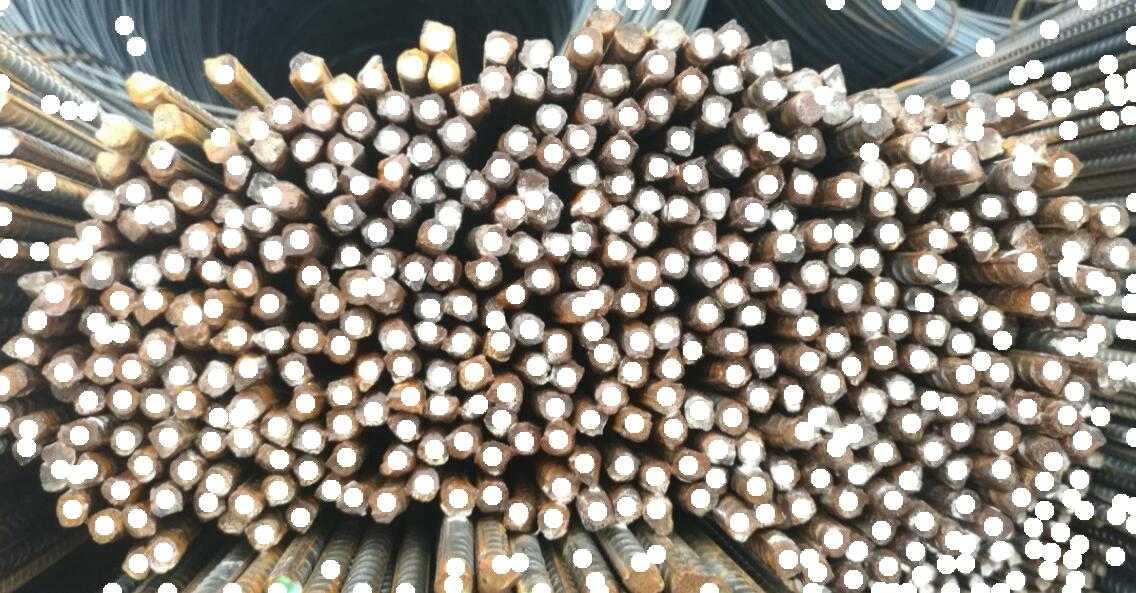2018-09-04 17:14:27 sy20173081277 阅读数 1564
• ###### [谭科]Excel函数视频课程（第1季）-初级

包含：Excel函数基础、求和函数、计数函数、条件求和函数、查找引用函数、文本函数、日期函数、数据库函数等。

18647 人正在学习 去看看 谭科

基于图像处理的目标数量的自动计数方法可以对采集到的图像进行处理来实现自动计数，也可以先用采集到的图像减去背景图像从而得到一个差值图像，然后对差值图像进行处理实现计数。无论哪种方式，都需要首先进行目标和背景的分割，并将分割出来的前景进行粘连分割处理，计数的精确度也受到这一过程重要的影响。

图像分割方法大致可以分为：基于区域的分割方法侧重于利用区域内特征的相似性；基于边缘检测的分割方法利用在区域边缘上的像素灰度值的变化比较剧烈这一实际来解决图像分割问题；区域与边缘检测相结合的分割方法；基于神经网络的分割方法利用神经网络在细粒度特征提取方面的优势，可以挖掘图像本身深层次的细节特征从而更好地分割图像；基于模糊集理论的分割方法包括模糊阈值分割方法、模糊聚类分割方法和模糊连接度分割方法等。

在进行分割之前，关于彩色图像和灰度图像的处理，一般如果原始图像为彩色图像则转化为灰度二值图像，然后在进行分割处理，如果是灰度图则可以直接利用上述的方法进行分割处理。

关于图像计数方面，国内外都进行了大量的研究，比较常见的有，细化法，连通域法，像素点分布法等

2018-04-17 23:04:54 u010030977 阅读数 2813
• ###### [谭科]Excel函数视频课程（第1季）-初级

包含：Excel函数基础、求和函数、计数函数、条件求和函数、查找引用函数、文本函数、日期函数、数据库函数等。

18647 人正在学习 去看看 谭科

# 波点壁纸中圆点的计数

### 解决思路：

• 转换色彩空间至HSV
• 根据H通道进行阈值分割
• 中值滤波去噪
• 腐蚀操作去除孤立的点
• 检测所有图形的轮廓，并计数

1、工业级的图像处理库：Opencv
2、PIL
3、Skimage

## 转换色彩空间至HSV

``````@adapt_rgb(each_channel)
def median_each(image):
return sfr.median(image, disk(3))

img_path = '波点壁纸.jpg'

dst = median_each(img_data)
plt.figure('filters',figsize=(20,20))

plt.subplot(121)
plt.title('origin image')
plt.imshow(img_data)

plt.subplot(122)
plt.title('Smoothed image')
plt.imshow(dst)``````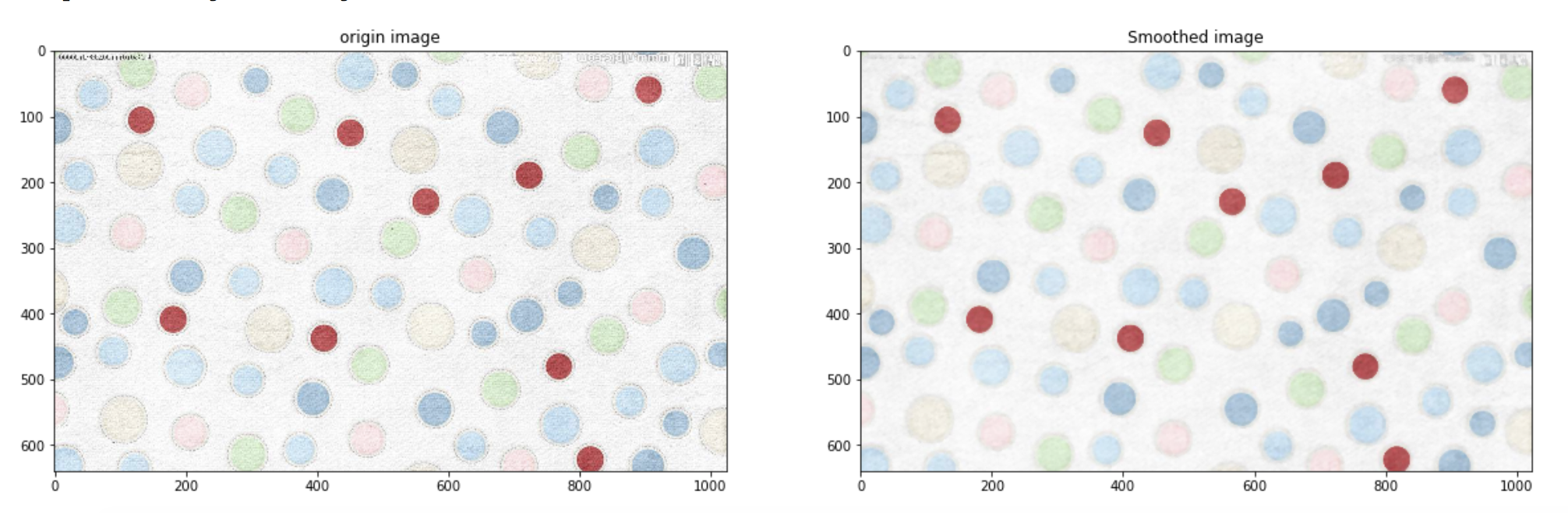## 根据H通道进行阈值分割

``````img_hsv_1 = color.rgb2hsv(dst)
plt.imshow(img_hsv_1,plt.cm.hsv)``````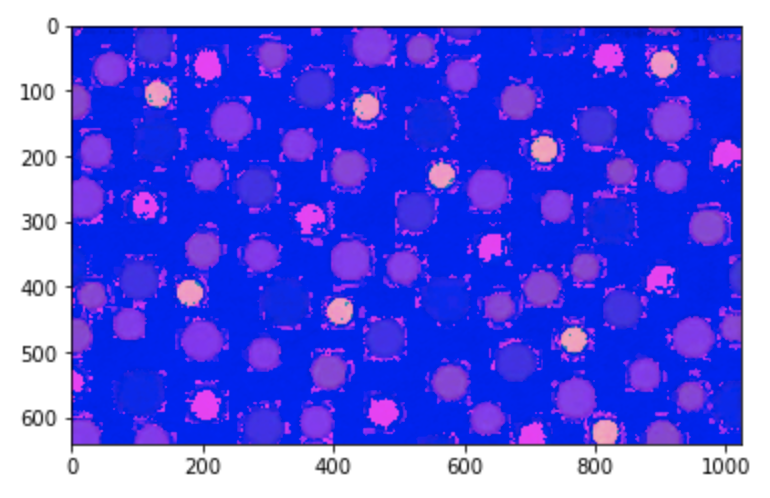## 中值滤波去噪

``````# H通道直方图
img = img_hsv_1[:,:,0]
plt.figure("hist")
arr=img.flatten()
plt.hist(arr, bins=256, normed=1,facecolor='r',edgecolor='r',hold=1)
plt.show()``````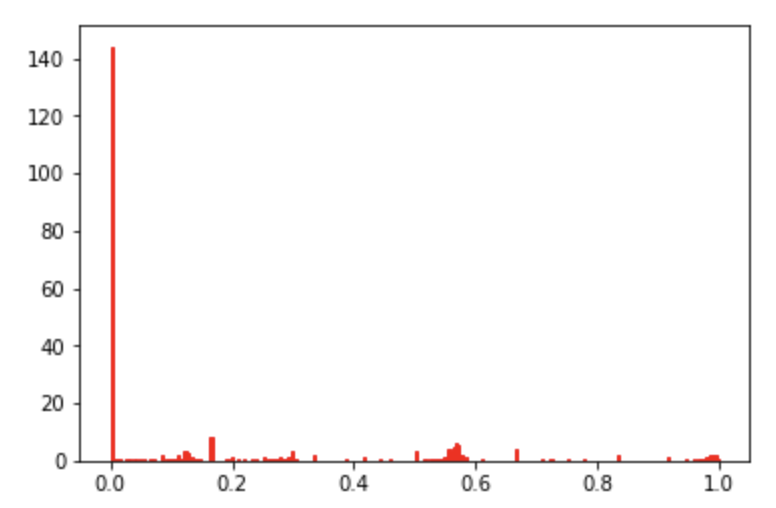``````# 手动查看鼠标对应H值
%matplotlib qt5
loop_num = 5
plt.imshow(img)
pos=plt.ginput(loop_num)
for i in range(loop_num):
x,y = int(pos[i]),int(pos[i])
print '第%d个点击的 x,y：' % int(i+1) ,'(', x , y,')'
print '对应的H值为：',img[x,y],'\n'``````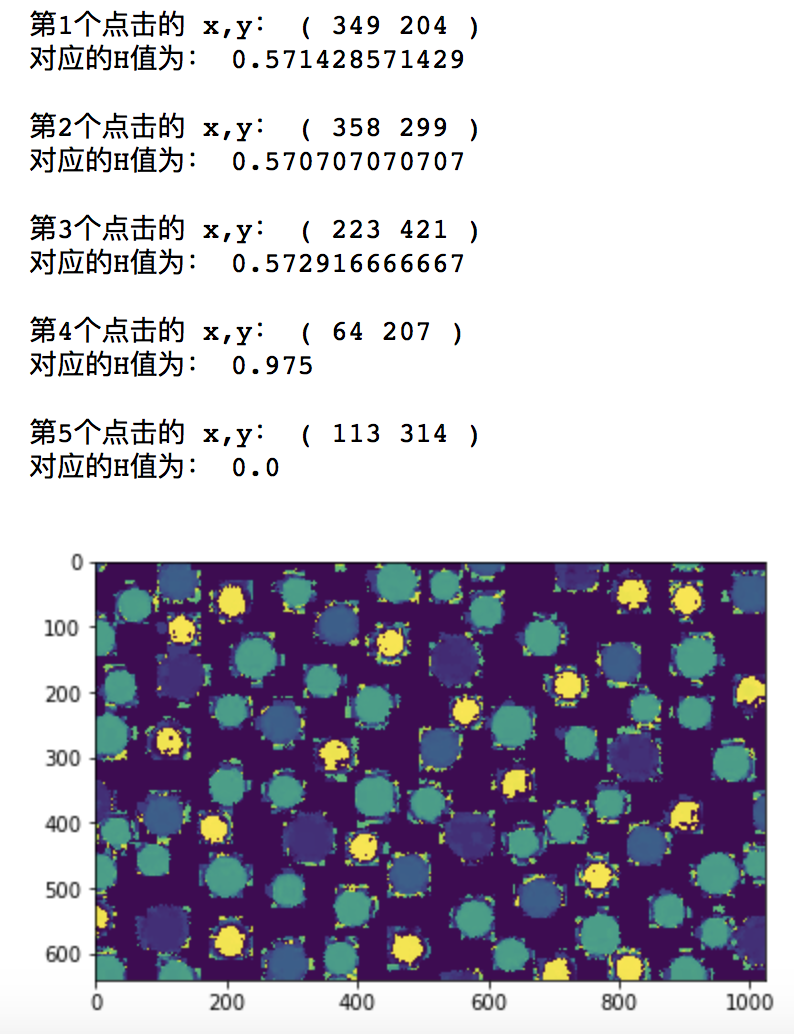``````# 手动选取阈值
%matplotlib inline
rows,cols=img.shape
labels=np.zeros([rows,cols])
for i in range(rows):
for j in range(cols):
if(img[i,j]<0.53 or img[i,j]>0.60):# 0.53 0.65
labels[i,j]=0
else:
labels[i,j]=1
shuffle =  color.rgb2gray(labels)
io.imshow(shuffle)``````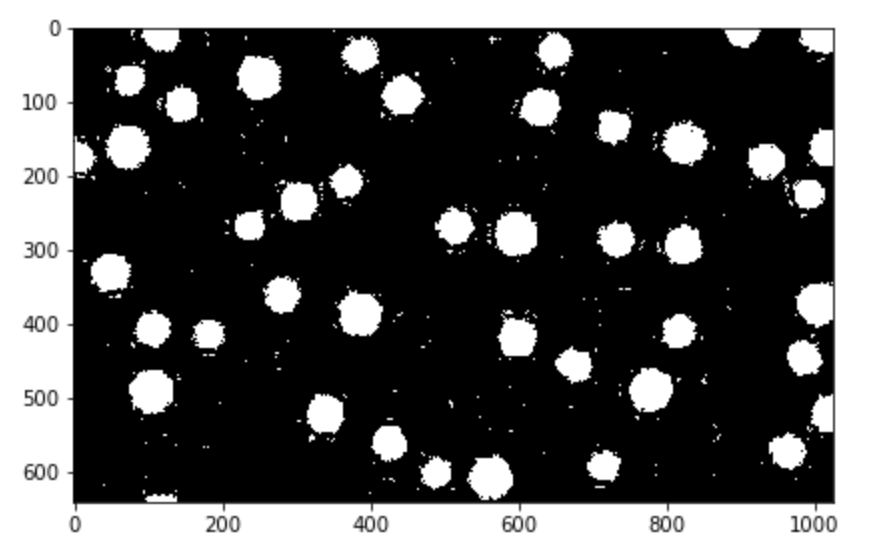## 中值滤波去噪

``````# 中值滤波去噪
shuffle_ = sfr.median(shuffle, disk(5))
new_img = color.rgb2gray(shuffle_)
io.imshow(new_img)``````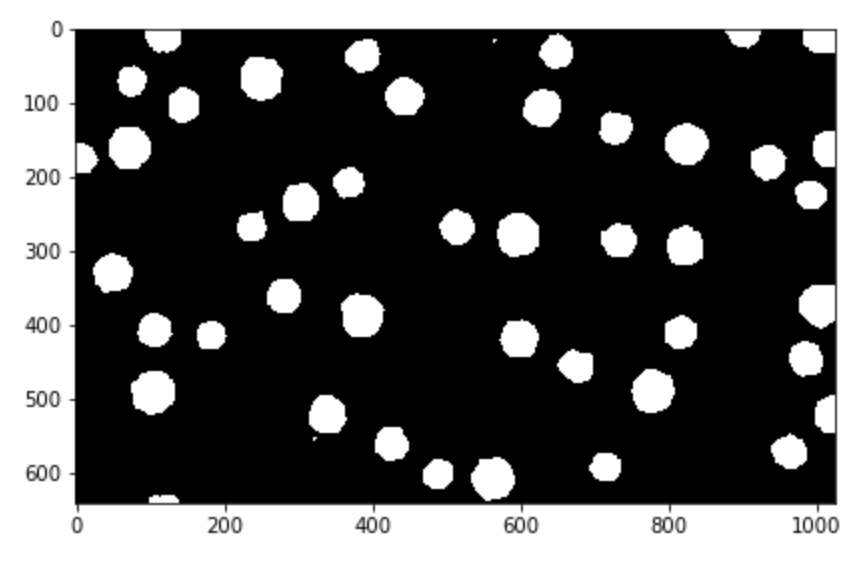## 腐蚀操作去除孤立的点

``````# 腐蚀操作，去除孤立的点
new_img_fat = sm.erosion(new_img,sm.square(5))

plt.figure('filters',figsize=(20,20))

plt.subplot(131)
plt.title('origin image')
io.imshow(new_img)

plt.subplot(132)
plt.title('fat image')
io.imshow(new_img_fat)

plt.subplot(133)
plt.title('fat - orgin')
io.imshow(new_img_fat - new_img)``````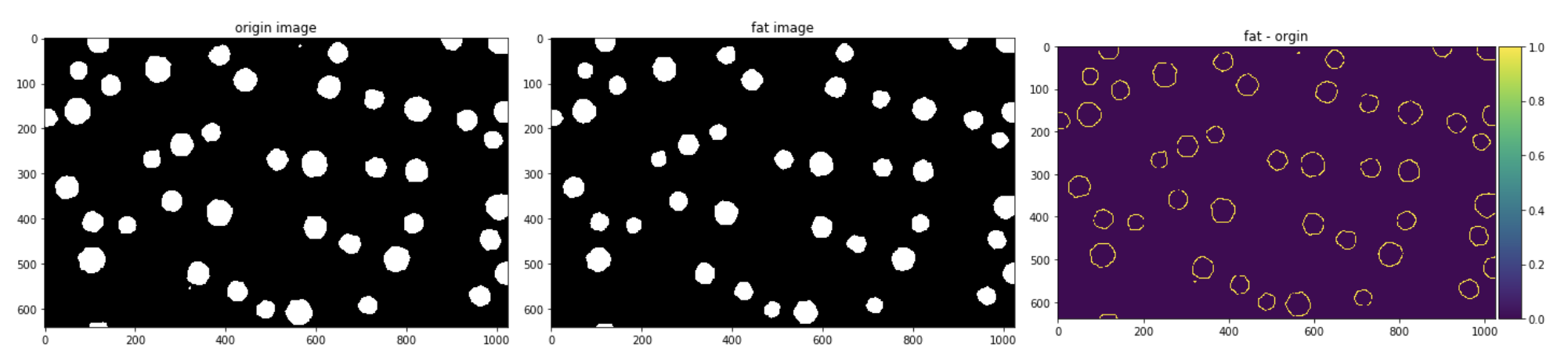## 检测所有图形的轮廓，并计数

``````#检测所有图形的轮廓
contours = measure.find_contours(new_img_fat, 0.5)

#绘制轮廓
fig, (ax0,ax1) = plt.subplots(1,2,figsize=(16,16))
ax0.imshow(new_img_fat,plt.cm.gray)
ax1.imshow(new_img_fat,plt.cm.gray)

for n, contour in enumerate(contours):
ax1.plot(contour[:, 1], contour[:, 0], linewidth=2)
ax1.axis('image')
ax1.set_xticks([])
ax1.set_yticks([])
plt.show()
print '总共有多少个⭕️：',len(contours)``````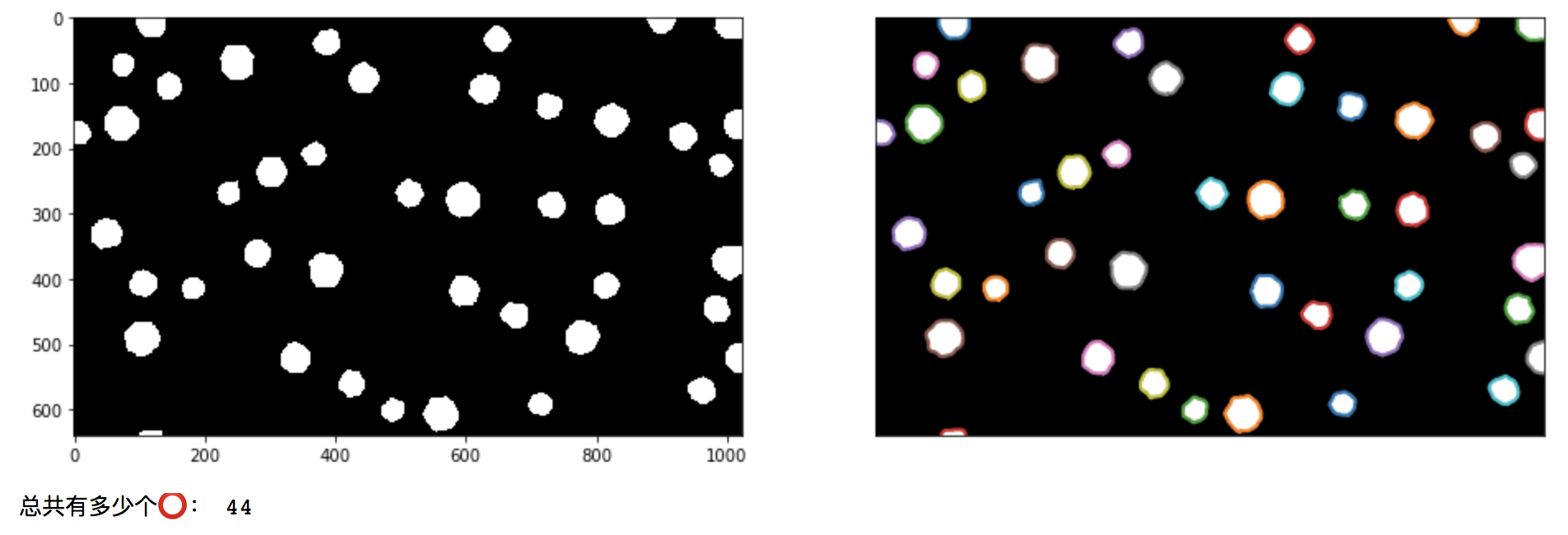## 总结

2016-12-12 21:37:25 LearningAlways 阅读数 1842
• ###### [谭科]Excel函数视频课程（第1季）-初级

包含：Excel函数基础、求和函数、计数函数、条件求和函数、查找引用函数、文本函数、日期函数、数据库函数等。

18647 人正在学习 去看看 谭科

``````dev_clear_window()
*读取图片
get_image_size (Image, Width, Height)
*自动阈值分割
bin_threshold (Image, Region)
*分割后选择的是所需的背景,所以求反一次
complement (Region, RegionComplement)
dev_display(Image)
* dev_display(RegionComplement)
*做一次闭运算
closing_circle(RegionComplement, RegionClosing, 3.5)
*空洞填充
fill_up_shape (RegionClosing, RegionFillUp, 'area', 1, 100)
*欧式距离函数的距离变换
distance_transform (RegionFillUp, DistanceImage, 'octagonal', 'true', Width, Height)
*int4转byte
convert_image_type (DistanceImage, ImageConverted, 'byte')
*图像取反
invert_image (ImageConverted, ImageInvert)
*增加对比度
scale_image_max (ImageInvert, ImageScaleMax)
*高斯平滑处理
gauss_image (ImageScaleMax, ImageGauss, 11)
*分水岭算法
watersheds_threshold (ImageGauss, Basins, 10)
*计数统计为29个
count_obj (Basins, Number)
*发现有小区域面积干扰,重新选择区域
select_shape (Basins, SelectedRegions, 'area', 'and', 5267.61, 119050)
*获取到正确的数目25
count_obj (SelectedRegions, Number1)``````

2019-03-26 15:04:29 weixin_40920848 阅读数 1271
• ###### [谭科]Excel函数视频课程（第1季）-初级

包含：Excel函数基础、求和函数、计数函数、条件求和函数、查找引用函数、文本函数、日期函数、数据库函数等。

18647 人正在学习 去看看 谭科

1. 图像格式的转换，及RGB图像转化为GRAY图像，这步大家都了解的；
2. 然后进行图像的分割，中间会利用一些形态学处理方式，主要思想就是先进行膨胀扩大细胞边缘，然后进行腐蚀取反以确定细胞核的位置，之后采用分水岭算法将连接的细胞分割开；
3. 对图像进行高斯滤波使图像变得模糊，细胞核相对于细胞边缘颜色较深，突出显示细胞核位置；
4. 对图像进行锐化获得细胞的轮廓位置信息并进行边沿去除操作；
5. 通过中值滤波之后对检测到的细胞进行编号。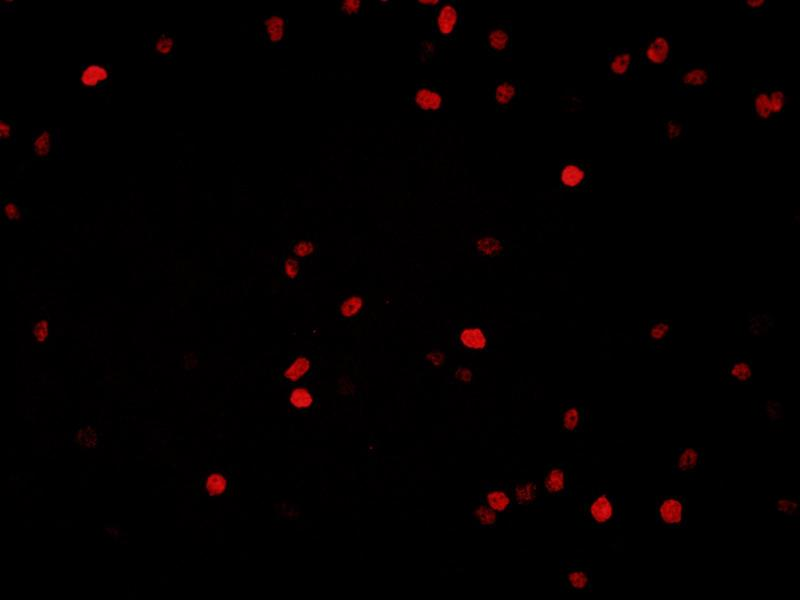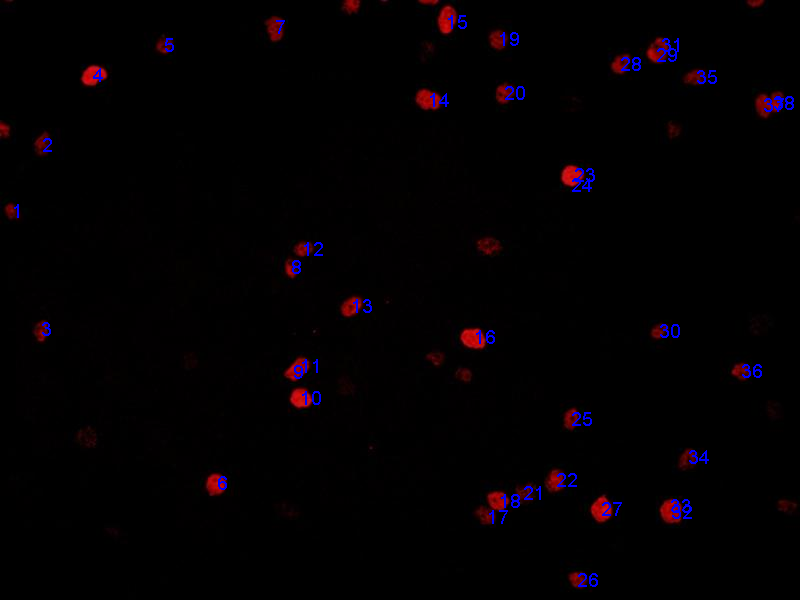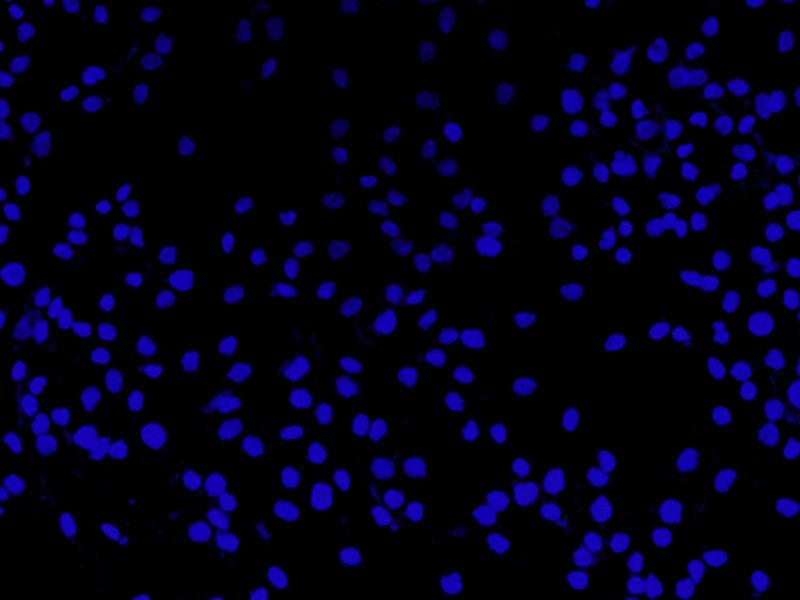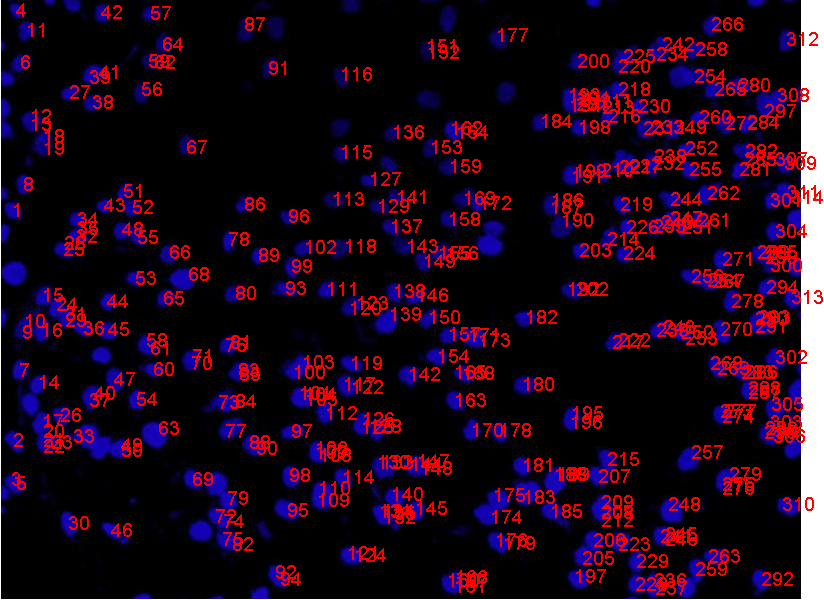``````clc;close all;clear all;
subplot(2,3,1);imshow(origin_img);title('原始图像');
[m,n]=size(origin_img);
img=rgb2gray(origin_img);%灰度
B=origin_img(:,:,3);
B_gray=im2double(B);
level=graythresh(B_gray);
B_bw=im2bw(B_gray,level);
show_img1=B_bw;
subplot(2,3,2);imshow(show_img1);title('灰度图像');
D=-bwdist(~show_img1);
Ld=watershed(D2);
Water_splited=show_img1;
Water_splited(Ld==0)=0;
show_img1=Water_splited;
subplot(2,3,3);imshow(show_img1);title('分割后图像');
sigma=3;
gausFilter=fspecial('gaussian',[5,5],sigma);
Mod=imfilter(img,gausFilter,'replicate');%高斯滤波
subplot(2,3,4);imshow(Mod);title('模糊图像');
lamda=1;
img2=(img-lamda*Mod)/(1-lamda);
subplot(2,3,5);imshow(uint8(img2));title('锐化后图像');%锐化后图像
img3=imclearborder(img2,8);
subplot(2,3,6);imshow(uint8(img3));title('去除边缘图像');%锐化后图像
% % BW = imregionalmin(img3,8);%最小连通区域
% P=BW+5;
% % img4 = bwareaopen(img3,P,8);%删除小于P后的图像
% imwrite(uint8(img2),'leansharpe.jpg');
K=imnoise(img3,'salt & pepper',0.005);%加入椒盐噪声
n2=12;%中值滤波的模板的大小
I=im2bw(K);
Y3=medfilt2(K,[n2 n2]); %调用系统函数进行中值滤波，n2为模板大小
[L,num] = bwlabel(Y3,8);
STATS1=regionprops(L,'Perimeter');
ahe=size(STATS1);
figure(2);imshow(origin_img);
m1=ahe(1,1);
m=zeros(2,m1);
for i=1:m1
% 计算目标区域中心，用于显示编号的位置
[p,q]=find(L==i);
temp=[p,q];
[x,y]=size(temp);
m(1,i)=sum(p)/x;
m(2,i)=sum(q)/x;
end
for i=1:m1
figure(2);
text(m(2,i),m(1,i),int2str(i),'FontSize',12,'color','red','LineWidth',10)
end
num``````

2018-04-18 00:16:43 u010030977 阅读数 3705
• ###### [谭科]Excel函数视频课程（第1季）-初级

包含：Excel函数基础、求和函数、计数函数、条件求和函数、查找引用函数、文本函数、日期函数、数据库函数等。

18647 人正在学习 去看看 谭科

# 存在接壤现象的细胞计数

### 解决思路：

• 进行锐化和平滑滤波操作
• 根据灰度图进行阈值分割
• 形态学处理
• 分水岭算法
• 修正

1、工业级的图像处理库：Opencv
2、PIL
3、Skimage

## 进行锐化和平滑滤波操作

``````# 锐化+平滑滤波操作
img_path1 = '细菌图.jpg'

from PIL import Image, ImageFilter
im = Image.open(img_path1)
# 没找到skimage的锐化api...所以用了PIL的
im.filter(ImageFilter.SHARPEN).save('细菌图_temp.jpg')

img = color.rgb2gray(sharpen_data)
dst = median_each(img)

plt.figure('filters',figsize=(14,14))

plt.subplot(121)
plt.title('origin image')
plt.imshow(orgin_img)

plt.subplot(122)
plt.title('sharpen and Smoothed image')
plt.imshow(dst,plt.cm.gray)``````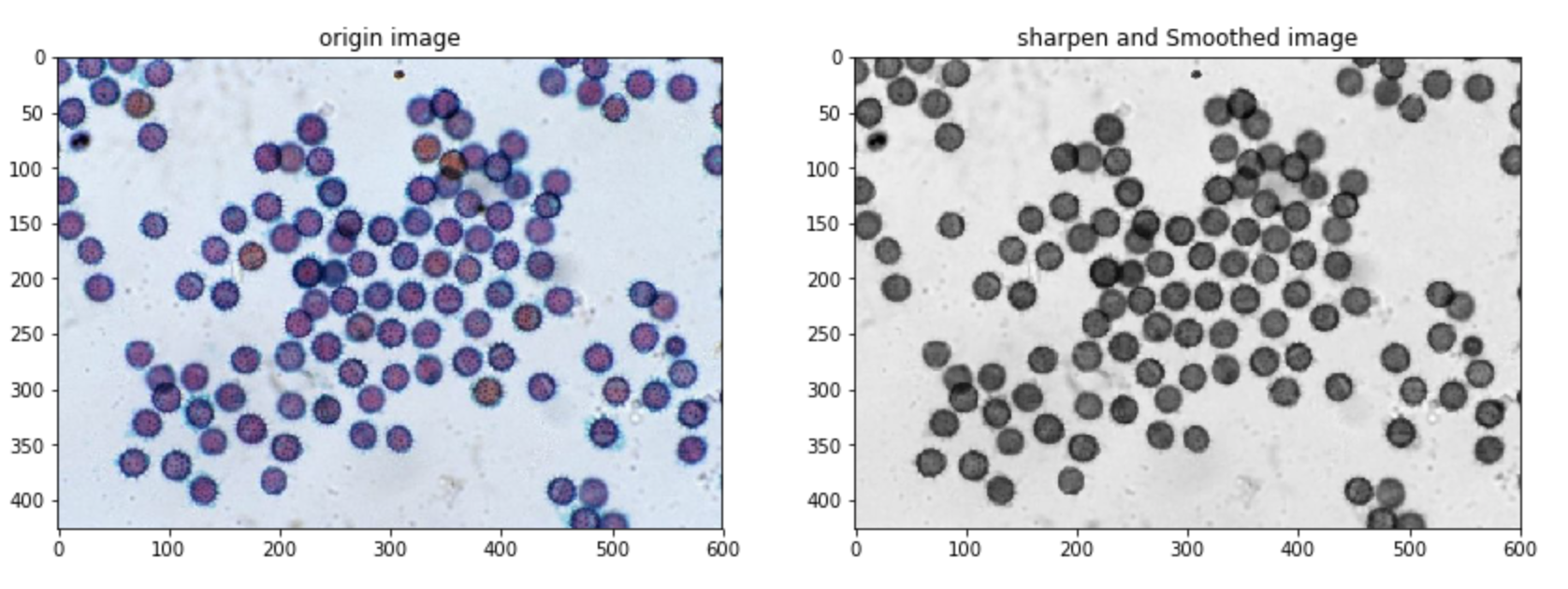## 根据灰度图进行阈值分割

``````# 直方图信息
plt.figure("hist")
arr=dst.flatten()
plt.hist(arr, bins=256, normed=1,facecolor='b',edgecolor='b',hold=1)
plt.show()``````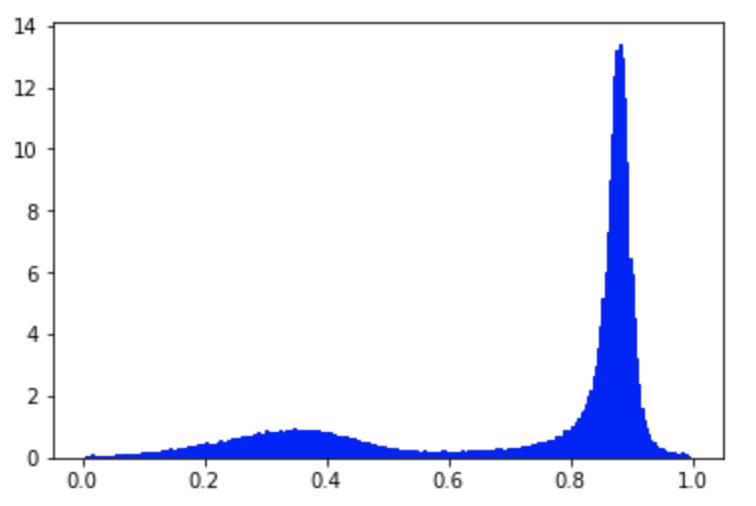``````# 手动查看鼠标对应H值
%matplotlib qt5
loop_num = 5
plt.imshow(dst,plt.cm.gray)

pos=plt.ginput(loop_num)
for i in range(loop_num):
x,y = int(pos[i]),int(pos[i])
print '第%d个点击的 x,y：' % int(i+1) ,'(', x , y,')'
print '对应的灰度值为：',dst[x,y],'\n'``````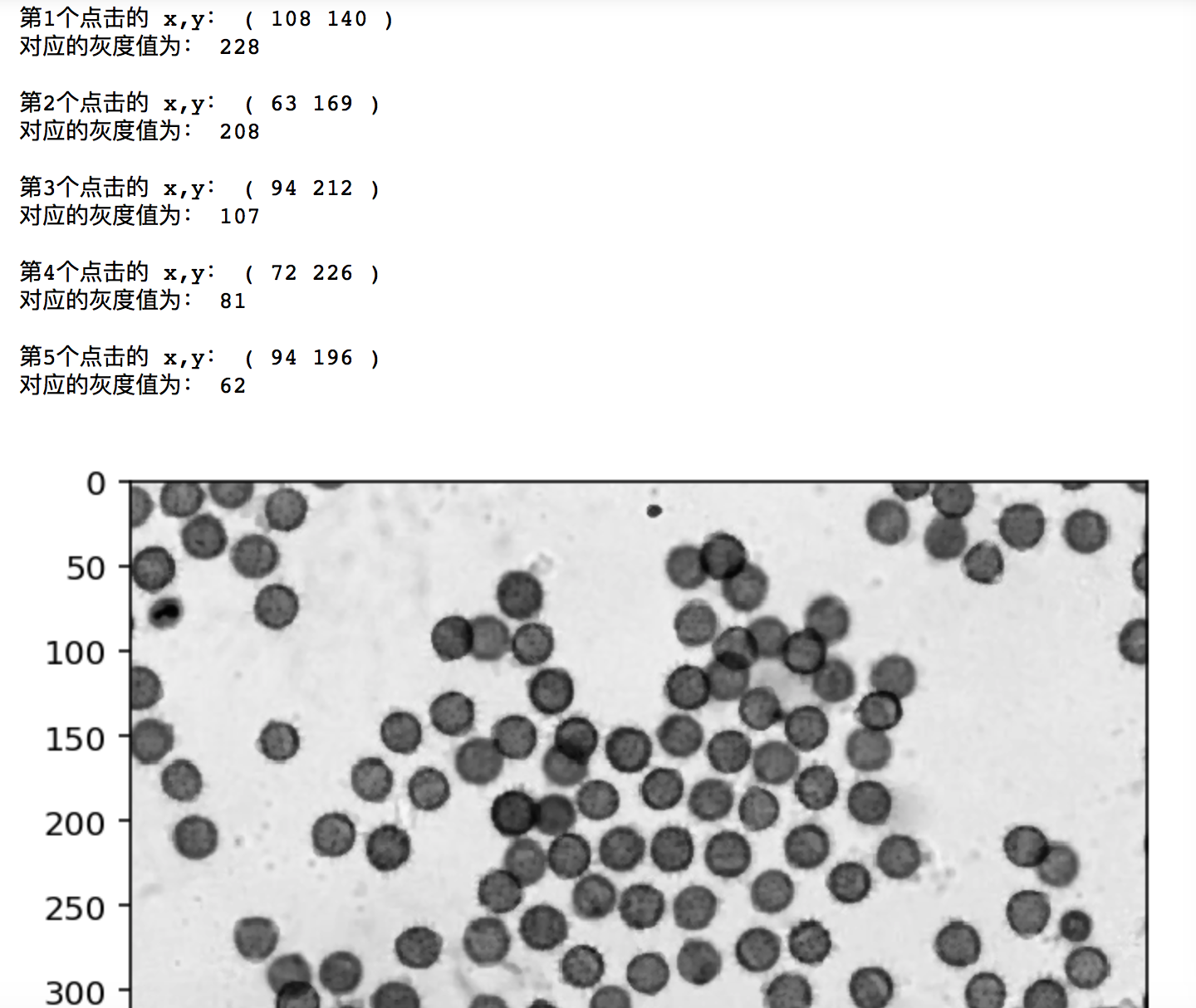``````# 手动选取阈值
%matplotlib inline
rows,cols=dst.shape
labels=np.zeros([rows,cols])
for i in range(rows):
for j in range(cols):
if(dst[i,j] < 135):
labels[i,j]=1
else:
labels[i,j]=0
io.imshow(labels)``````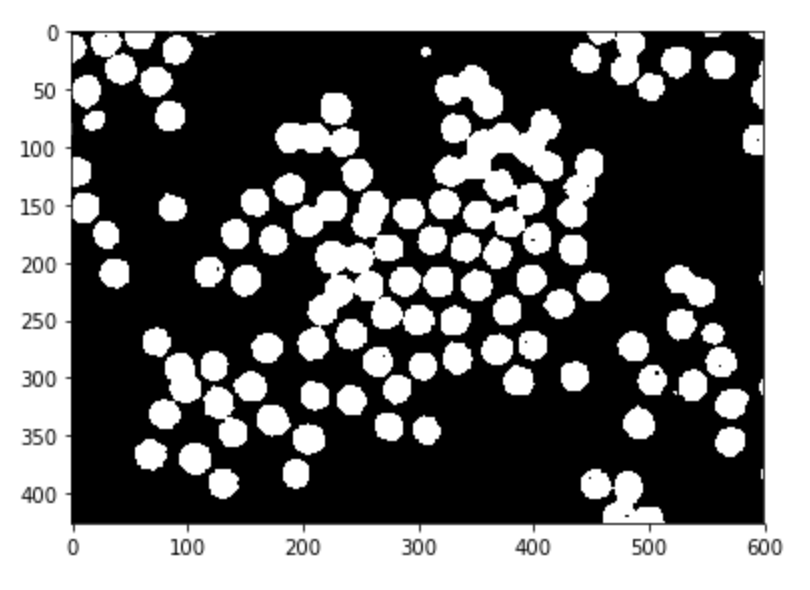## 形态学处理

``````# 膨胀,开操作
labels= sm.dilation(labels,sm.square(3))
labels = sm.opening(labels,sm.disk(5))``````

## 分水岭算法

``````from scipy import ndimage as ndi
# labels = dst
distance = ndi.distance_transform_edt(labels) #距离变换
# min_distance：最小的像素在2×min_distance + 1区分离（即峰峰数至少min_distance分隔）。找到峰值的最大数量，使用min_distance = 1。
# exclude_border：不排除峰值在图像的边界
# indices：False会返回和数组相同大小的布尔数组，为True时，会返回峰值的坐标
local_maxi =feature.peak_local_max(distance, exclude_border = 0,min_distance = 12,indices=False,
footprint=np.ones((10, 10)),labels=labels) #寻找峰值
markers = ndi.label(local_maxi) #初始标记点

fig, axes = plt.subplots(nrows=2, ncols=2, figsize=(12, 12))
axes = axes.ravel()
ax0, ax1, ax2, ax3 = axes

ax0.imshow(labels, cmap=plt.cm.gray)#, interpolation='nearest')
ax0.set_title("Original")
ax1.imshow(-distance, cmap=plt.cm.jet, interpolation='nearest')
ax1.set_title("Distance")
ax2.imshow(sm.dilation(markers,sm.square(10)), cmap=plt.cm.spectral, interpolation='nearest')
ax2.set_title("Markers")
ax3.imshow(label_, cmap=plt.cm.spectral, interpolation='nearest')
ax3.set_title("Segmented")

for ax in axes:
ax.axis('off')

fig.tight_layout()
plt.show()
``````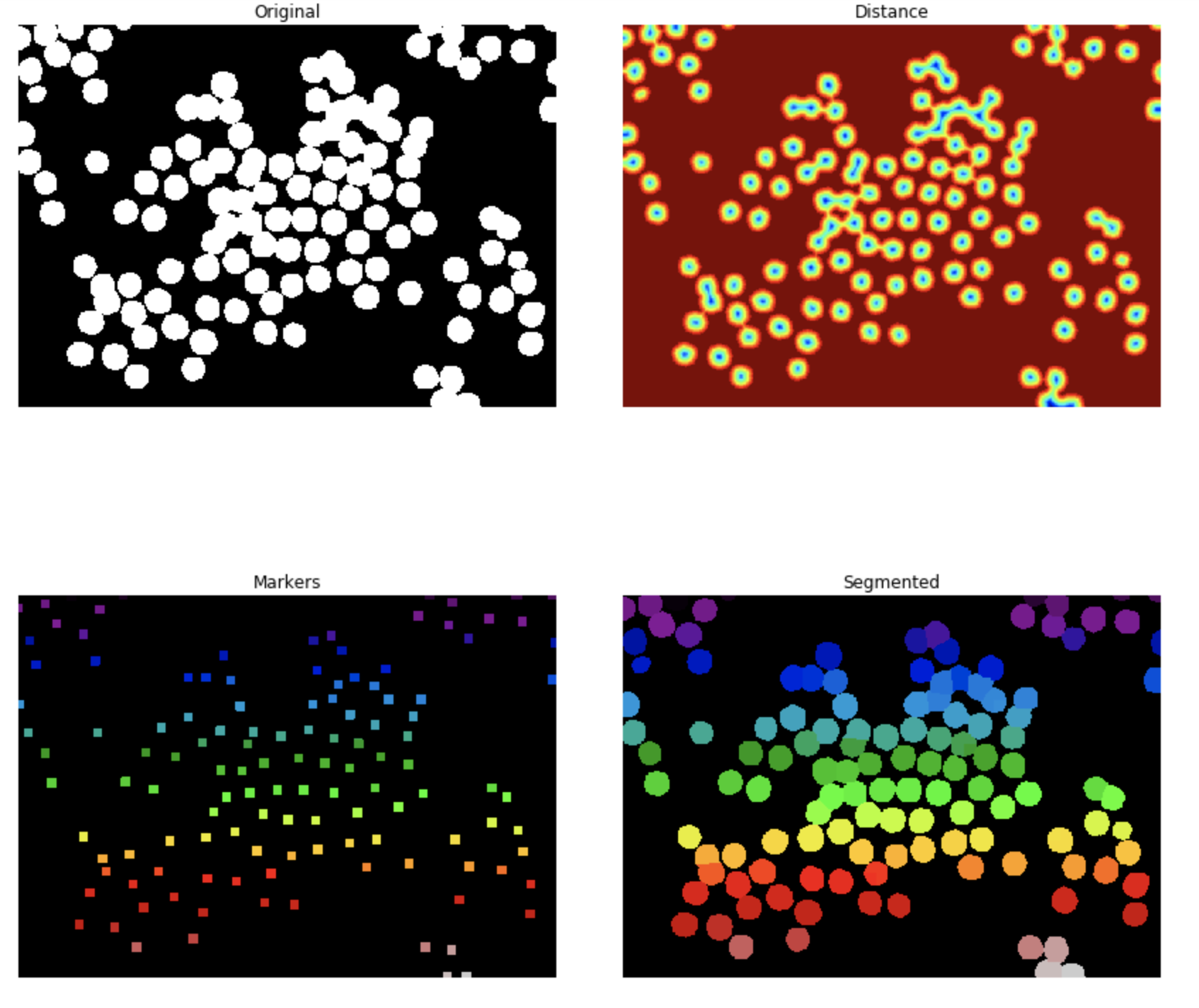## 修正

``````# 修正
import math
err = []
for i in range(x_y.shape):
h1,w1 =  x_y[i],x_y[i]
if i in err:
continue
for j in range(i+1,x_y.shape):
h2,w2 =  x_y[j],x_y[j]
ab = math.sqrt(math.pow(abs(h2-h1), 2) + math.pow(abs(w2-w1), 2))
if ab <= 10:
#             print 'error:' , x_y[i],' and ', x_y[j],'i,j = ',i,j
err.append(j)
new_x_y = []
for i in range(len(x_y)):
if i not in err:
new_x_y.append(x_y[i])
print '一共有',len(new_x_y),'个圈'``````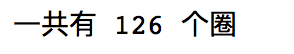## 本思路在钢筋图像上的效果：